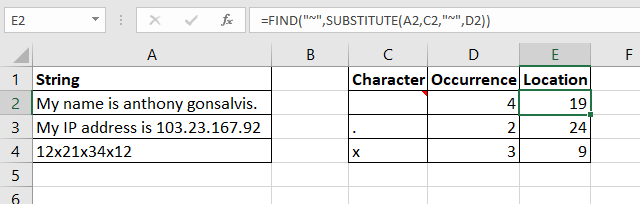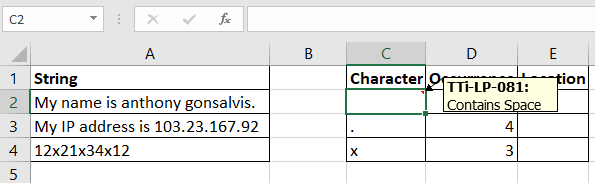# Find Occurrence of TextIn this article, we will learn Find Occurrence of Text in Excel.

Scenario:

To find the first occurrence of a character in Excel we use the FIND of SEARCH function in Excel. But if we want to find the second occurrence of a character in excel, both functions fail to do so. They need help to find the second, third or Nth occurrence of a character in Excel.

Excel Formula

 = SEARCH ( "~" , SUBSTITUTE ( string , character , "~" , occurrence ) )

"~" : This is a special character that will not appear in the given string. You can use any character or symbol that you are sure will not appear in the string you are going to search.

String : This is the string in which you want to search for the occurrence of character.

Character: It is the character whose occurrence you want to search in the string.

Occurrence: It is the number of the occurrence of the character in the string.

Example :

All of these might be confusing to understand. Let's understand how to use the function using an example. Find the Second, Third and Nth Occurrence of Given Characters in the Strings.Here we have some strings in range A2:A4. In cell C2, C3, and C4 we have mentioned the characters that we want to search in the strings. In D2, D3, and D4 we have mentioned the occurrence of the character. In the adjacent cell I want to get the position of these occurrences of the characters.

Write this formula in the cell E2 and drag down.

 =SEARCH("~", SUBSTITUTE(A2,C2,"~",D2))

This returns the exact positions (19) of the mentioned occurrence (4) of the space character in the string.How does it work?

The technique is quite simple. As we know the SUBSTITUTE function of Excel replaces the given occurrence of a text in string with the given text. We use this property.

So the formula works from inside.

SUBSTITUTE(A2,C2,"~",D2): This part resolves to SUBSTITUTE("My name is anthony gonsalvis."
," ","~",4). Which ultimately gives us the string "My name is anthony~gonsalvis."

Note that the fourth occurrence of space is replaced with "~". I replaced space with "~" because I am sure that this character will not appear in the string by default. You can use any character that you are sure will not appear in the string. You can use the CHAR function to insert symbols.

Now the SEARCH function has SEARCH("~","My name is anthony~gonsalvis."). Now that the character "~" appears only once at the position 19, we get our answer as 19.

Similarly all the formulas are solved.

If you want to use a case sensitive search then use the FIND function instead of SEARCH.

 =FIND("~", SUBSTITUTE(A2,C2,"~",D2))

How to find occurrence of text in a column through Conditional Formatting?

Hope this article about Find Occurrence of Text in Excel is explanatory. Find more articles on calculating values and related Excel formulas here. If you liked our blogs, share it with your friends on Facebook. And also you can follow us on Twitter and Facebook. We would love to hear from you, do let us know how we can improve, complement or innovate our work and make it better for you. Write to us at info@exceltip.com.

Related Articles :

How to Find Nth Occurrence in Excel : How do we LOOKUP second match or 3rd or nth? In this article, we will learn, how to get the Nth occurrence of a value in range. We don't use the normal lookup techniques. To find Nth occurrence

How to do Running Count of Occurance in Excel : Count of running occurrence, what we mean by that. Actually, we will try to get occurance of some text or value in a range by its position. For example, on first occurrence of “A”, we will tag it as 1, on second as 2, on third occurrence 3

How to Retrieve Every Nth Value in a Range in Excel : To retrieve every Nth value from a range in Excel, we use the INDEX and ROW function together. This formula gets all the occurrences of from the given range.

Find nth largest with criteria & nth smallest with criteria in excel : while working with numbers in data numbers, sometimes given a condition i.e. when we need to look up for the 2nd highest value given . You can perform the solution to this problem easily using the excel functions as explained below.

Popular Articles :

How to use the IF Function in Excel : The IF statement in Excel checks the condition and returns a specific value if the condition is TRUE or returns another specific value if FALSE.

How to use the VLOOKUP Function in Excel : This is one of the most used and popular functions of excel that is used to lookup value from different ranges and sheets.

How to use the SUMIF Function in Excel : This is another dashboard essential function. This helps you sum up values on specific conditions.

How to use the COUNTIF Function in Excel : Count values with conditions using this amazing function. You don't need to filter your data to count specific values. Countif function is essential to prepare your dashboard.

Terms and Conditions of use

The applications/code on this site are distributed as is and without warranties or liability. In no event shall the owner of the copyrights, or the authors of the applications/code be liable for any loss of profit, any problems or any damage resulting from the use or evaluation of the applications/code.